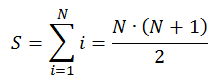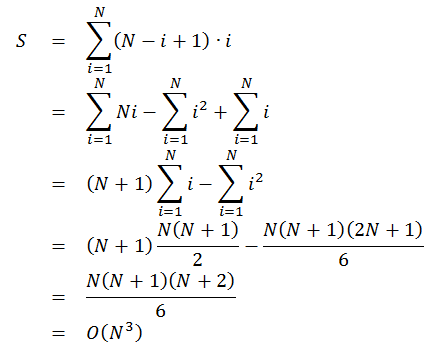# Maximum Sum Subarray

by Zoran Horvat

## Problem Statement

Given an array with N integers, write a function which finds subarray consisting of contiguous elements of the array such that sum of elements of the subarray is maximized. If two subarrays have the same sum, function should produce the shorter one. If two subarrays with same sum have the same length, function should produce the one that starts at lower array index.

Example: If array is 1 2 -3 3, then largest sum subarray has length 1 and consists only of the last array element 3. If array is 1 2 -3 3 -2 4, then last three numbers (3, -2 and 4) are the largest sum subarray, with sum 5.

## Problem Analysis

As the first step towards finding an efficient solution to this problem, we might ask how many possible subarrays one array has. This would give us an idea how to proceed in looking for the best subarray. For array consisting of N numbers, there are total of N subarrays of length 1; N-1 subarrays of length 2; N-2 subarrays of length 3; and so on, ending with one subarray of length N. Number of all subarrays of any length is then:This number is not too large, but number of array elements that we touch varies with length of the subarray. In particular, we are summing subarrays of length 1 total of N times, subarrays of length 2 total of N-1 times, and so on. So total number of touches made to array elements in order to find maximum subarray by exhausting all options is:In this derivation we have used equations for arithmetic sequence sum and for sum of squares, which are explained in exercises Sum of First N Numbers and Sum of Squares of First N Numbers .

The result indicates that problem can be solved by force only for reasonably small arrays. We could iterate through all subarrays and take their sums, looking for the one that produces largest sum. Here is the brute force solution:

``````function MaxSumSubarrayForce(a, n, start out, len out)
-- a - Array
-- n - Length of the array
-- start - On output starting position of largest subarray
-- len - On output length of largest subarray
-- Returns sum of the largest subarray
begin

start = 0
len = 1
sum = a

for curLen = 1 to n
begin

for curStart = 1 to n - curLen + 1
begin

curSum = 0
for i = 0 to curLen - 1
curSum = curSum + a[curStart + i]

if curSum > sum then
begin
start = curStart
len = curLen
sum = curSum
end

end

end

return sum

end
``````

This function gradually increases the desired length of the subarray. For any given length, it iterates through all subarrays of that length. Whenever a higher sum is reached, current subarray is remembered. The one that prevails is the overall winner.

Now that we have a dummy solution in hand, we can ask whether there is a better one? In order to enhance running speed of the solution, we could investigate what happens if an array is extended by one element. Suppose that we have solved the problem for the array with N-1 elements and now the Nth element is added at the end of the array. New best solution is either the previous best, or some subarray that ends in position N-1 extended by the new element. This solution requires two solutions for the array with N-1 elements: overall best subarray, and best subarray strictly ending in position N-1. With these two pieces of information, it is quite easy to determine next best subarray. Off course, this solution requires an initial state: we are starting with an array of length 1 and it is the best solution itself. Below is the function which finds the largest sum subarray.

``````function MaxSumSubarray(a, n, start out, len out)
-- a - Array
-- n - Length of the array
-- start - On output starting position of largest subarray
-- len - On output length of largest subarray
-- Returns sum of the largest subarray
begin

start = 0
len = 1
int sum = a

curStart = 0
curLen = 1
curSum = a

for i = 2 to n
begin
if a[i] >= curSum + a[i] then
begin
curStart = i
curLen = 1
curSum = a[i]
end
else
begin
curLen = curLen + 1
curSum = curSum + a[i]
end

if (curSum > sum) OR
(curSum = sum AND curLen < len) OR
(curSum = sum AND curLen = len AND curStart < start) then
begin
start = curStart
len = curLen
sum = curSum
end

end

return sum

end
``````

Running time of this solution is O(N), which is significantly better than running time of the brute force implementation, which was O(N^3). This function is the variation of Kadane's algorithm for the maximum subarray problem.

## Implementation

Below is the complete implementation of console application in C# which inputs arrays and then produces their maximum subarrays. Note that there are slight differences compared to the function above, because arrays in C# are zero-based.

``````using System;

namespace MaximumSumSubarray
{

public class Program
{

{

Console.Write("Enter array length (0 to exit): ");
int n = int.Parse(Console.ReadLine());

int[] a = null;

if (n > 0)
{

a = new int[n];

Console.Write("Enter array elements (space-separated): ");
string[] elements =
Console
.Split(new char[] { ' ' }, StringSplitOptions.RemoveEmptyEntries);

for (int i = 0; i < n; i++)
a[i] = int.Parse(elements[i]);

}

return a;

}

static int MaxSumSubarray(int[] a, out int start, out int len)
{

start = 0;
len = 1;
int sum = a;

int curStart = 0;
int curLen = 1;
int curSum = a;

for (int i = 1; i < a.Length; i++)
{

if (a[i] >= curSum + a[i])
{
curStart = i;
curLen = 1;
curSum = a[i];
}
else
{
curLen++;
curSum += a[i];
}

if ((curSum > sum) ||
(curSum == sum && curLen < len) ||
(curSum == sum && curLen == len && curStart < start))
{
start = curStart;
len = curLen;
sum = curSum;
}

}

return sum;

}

static void PrintArray(int[] a, int start, int len, int sum)
{

for (int i = 0; i < a.Length; i++)
{
Console.Write("{0}{1}{2}",
i == start ? '[' : ' ',
a[i],
i == start + len - 1 ? ']' : ' ');
}

Console.WriteLine(" ({0})", sum);

}

static void Main(string[] args)
{

while (true)
{

int[] a = ReadArray();

if (a == null)
break;

int start = 0;
int len = 0;
int sum = MaxSumSubarray(a, out start, out len);

PrintArray(a, start, len, sum);

}

}

}
}
``````

## Demonstration

When application is run, it produces output like this:

```            ```
Enter array length (0 to exit): 4
Enter array elements (space-separated): 1 2 -3 3
1  2  -3  (3)
Enter array length (0 to exit): 4
Enter array elements (space-separated): 1 2 -2 3
[1  2  -2  3] (4)
Enter array length (0 to exit): 6
Enter array elements (space-separated): 1 2 -3 3 -2 4
1  2  -3 [3  -2  4] (5)
Enter array length (0 to exit): 6
Enter array elements (space-separated): 5 6 -10 7 -8 9
[5  6] -10  7  -8  9  (11)
Enter array length (0 to exit): 7
Enter array elements (space-separated): 5 6 -10 7 -8 9 2
[5  6] -10  7  -8  9  2  (11)
Enter array length (0 to exit): 0
```
```